Friday  December 3, 2021
 AmosWEB means Economics with a Touch of Whimsy!EQUILIBRIUM PRICE: The price that exists when a market is in equilibrium. In particular, the equilibrium price is the price that equates the quantity demanded and quantity supplied, which is termed the equilibrium quantity. Moreover, the equilibrium price is simultaneously equal to the both the demand price and supply price. In a market graph, like the one displayed here, the equilibrium price is found at the intersection of the demand curve and the supply curve. The equilibrium price is also commonly referred to as the market-clearing price.PERFECT COMPETITION, SHORT-RUN PRODUCTION ANALYSIS:

A perfectly competitive firm produces the profit-maximizing quantity of output that equates marginal revenue and marginal cost. This production level can be identified using total revenue and cost, marginal revenue and cost, or profit. Because a perfectly competitive firm faces a perfectly elastic demand curve, it efficiently allocates resources by equating price and marginal cost. In addition, the marginal cost curve above the average variable cost curve is the perfectly competitive firm's short-run supply curve.
Perfect competition is a market structure characterized by a large number of small firms producing identical products with perfect resource mobility and perfect knowledge. These conditions mean a perfectly competitive firm faces a horizontal, or perfectly elastic, demand curve. With this horizontal demand curve, marginal revenue is equal to average revenue, both of which are also equal to price.

Comparable to any profit-maximizing firm, a perfectly competitive firm produces the quantity of output in the short run that equates marginal revenue with marginal cost. At this production level, the firm cannot increase profit by changing the level of production. Because price is equal to marginal revenue, a perfectly competitive firm also produces the quantity of output that equates price and marginal cost, which is the essential condition for efficiency.

The profit-maximizing equality between price and marginal cost means that a perfectly competitive firm moves along its marginal cost curve in response to price changes. As such, the marginal cost curve, especially the upward-sloping portion that lies above the average variable cost curve, is the perfectly competitive firm's short-run supply curve. This conclusion provides a rationale for the positively-sloped market supply and the law of supply.

### Determining Output

A perfectly competitive firm is presumed to produce the quantity of output that maximizes economic profit--the difference between total revenue and total cost. This production decision can be analyzed in three different, but interrelated ways.
• Profit: The first is directly through the analysis of economic profit, especially using a profit curve that traces the level of economic profit for different levels of output.
• Total Revenue and Cost: A second is by comparing total revenue and total cost, commonly accomplished with total revenue and total cost curves.
• Marginal Revenue and Cost: The third, and perhaps most noted, way is by comparing marginal revenue and marginal cost, similarly achieved with marginal revenue and marginal cost curves.
The profit-maximization production decision facing a perfectly competitive firm can be analyzed using the exhibit below. This table presents revenue and cost information for Phil the zucchini grower, a hypothetical example of a perfectly competitive firm.
Profit Maximization,
Zucchini Style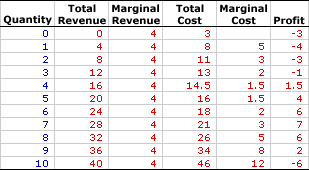• Quantity: The first column presents the quantity of output produced, ranging from 0 to 10 pounds of zucchinis.

• Total Revenue: The second column presents total revenue, ranging from a low of \$0 for no output to a high or \$40 for 10 pounds of zucchinis.

• Marginal Revenue: The third column presents marginal revenue, the change in total revenue due to a change in the quantity, which is equal to \$4 at each production level.

• Total Cost: The fourth column presents the total cost incurred by the firm in the production of this output, ranging from a low of \$3 for zero output (which is also fixed cost) to a high of \$46 for 10 pounds of zucchinis.

• Marginal Cost: The fifth column presents marginal cost, the change in total cost due to a change in the quantity, which declines from \$5, reaches a low of \$1.50, then rises until reaching \$12.

• Profit: The sixth column at the far right of the table is profit, the difference between total revenue in the second column and total cost in the third column. It begins at -\$3, falls to -\$4, rises to \$7, then falls to -\$6.
The choice facing Phil is to produce the quantity of zucchinis that maximizes profit. This can be easily identified using the far right "Profit" column. The quantity that generates the greatest level of economic profit is 7 pounds of zucchinis. This alternative can be highlighted by clicking the [Profit Max] button.

The production of 7 pounds of zucchinis results in \$28 of total revenue and \$21 of total cost, a difference of \$7. No other production level generates a greater economic profit. Producing one more pound of zucchinis or one less pound of zucchinis reduces profit to \$6.

While marginal revenue and marginal cost might not appear to be equal for the profit-maximizing 7 pounds of zucchini production (\$4 versus \$3), they really are. The reason is that the marginal numbers in the table actually represent discrete changes from one pound to the next. Reducing the size of the discrete change, say from 6.9999 pounds to 7 pounds, results in a marginal cost that is closer to \$4. At the limit of an infinitesimally small change, marginal cost is exactly \$4 and equal to marginal revenue.

While the equality between marginal revenue and marginal cost shows up better in a graph, the best practical method of identifying similar results, with a table of numbers, is to average the discrete changes on either side of the quantity. For example, the marginal cost AT the seventh pound of zucchini production is the average of the change from 6 to 7 (\$3) and from 7 to 8 (\$5), which is \$4.

### Total Curves

Total Revenue and Cost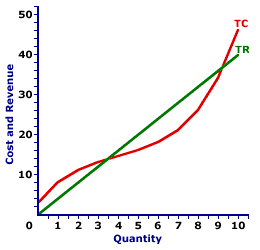The short-run production decision for a perfectly competitive firm can be graphically illustrated using total revenue and total cost curves, such as those displayed in the exhibit to the right. These total curves represent the total revenue and cost of zucchini production by Phil the zucchini grower.
• Total Revenue: The straight green line depicts the total revenue Phil receives from zucchini production. The line is straight, with a constant slope, because the price is constant at \$4.

• Total Cost: The curvy red line depicts the total cost Phil incurs in the production of zucchinis. The shape is based on increasing, then decreasing marginal returns.

• Profit: The vertical difference between these two lines is economic profit. If the total revenue line is above the total cost line in the middle of the diagram, economic profit is positive. If the total revenue line is below the total cost line at the far right and far left, economic profit is negative.
The key for Phil is to identify the production level that gives the greatest vertical distance between the total revenue and total cost curves in the middle of the diagram. This might not be evident by just looking at the exhibit, but it can be illustrated by clicking the [Profit Max] button. The output quantity identified is, once again, 7 pounds of zucchinis.

### Marginal Curves

Marginal Revenue and Cost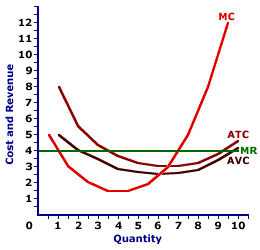Perhaps the most common method of identifying the profit-maximizing level of production for a perfectly competitive firm is using marginal revenue and marginal cost curves, such as those displayed in the this exhibit.
• Marginal Revenue: The horizontal green line (MR) is the marginal revenue Phil receives for each extra pound of zucchini production, which is constant and equal to \$4.

• Marginal Cost: The red U-shaped curve (MC) is the marginal cost Phil incurs in the production of zucchinis. The shape is based on increasing, then decreasing marginal returns.

• Average Cost: Two additional U-shaped curves included in the diagram (just for good measure) are average total cost (ATC) and average variable cost (AVC). These curves are helpful when identifying the level of economic profit or loss and the firm's short-run supply curve.
In this analysis, Phil needs to identify the quantity of output that achieves an equality between marginal revenue and marginal cost. This production can be highlighted by clicking the [Profit Max] button. The highlighted quantity is once again 7 pounds of zucchinis.

### Production Alternatives

Profit and Loss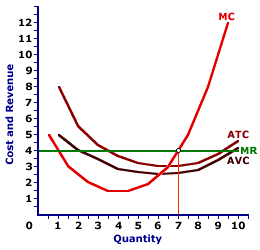A perfectly competitive firm faces three short-run production alternatives based on a comparison of price, average total cost, and average variable cost.
• P > ATC: Total revenue exceeds total cost and Phil receives a positive economic profit. In this case, Phil maximizes profit by producing the quantity of output that equates marginal revenue and marginal cost. This is the initial situation displayed in the graph.

• ATC > P > AVC: Total revenue falls short of total cost, meaning Phil incurs an economic loss (or negative economic profit). In spite of the loss, because the price exceeds average variable cost, Phil can maximize profit (minimize loss) by producing the quantity of output that equates marginal revenue and marginal cost. Phil receives enough revenue to cover ALL variable cost plus a portion of fixed cost. The loss from production is less than the loss from NOT producing, which is total fixed cost. This can be displayed by clicking the [\$2.60] button.

• P < AVC: Total revenue also falls short of total cost, and Phil incurs an economic loss (or negative economic profit). In this case Phil maximizes profit (that is, minimizes loss) by reducing the quantity of output to zero, or producing no output in the short run. By producing a positive quantity, Phil does not receive enough revenue to cover variable cost, let alone any fixed cost. The economic loss from producing is greater than the economic loss of NOT producing, which is total fixed cost. This can be illustrated by clicking the [\$2.00] button.

### Short-Run Supply Curve

Short-Run Supply Curve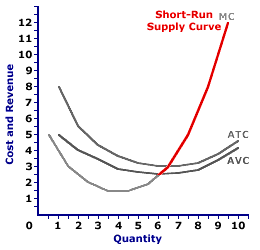A perfectly competitive firm's marginal cost curve that lies above the minimum of the average variable cost curve is its supply curve. This is highlighted in the exhibit to the right.

Because a perfectly competitive firm maximizes profit by producing the quantity of output that equates price and marginal cost it moves along its marginal cost curve in response to alternative prices.

This conclusion holds only if the firm is a perfectly competitive price taker. The marginal cost curve is a supply curve only because a perfectly competitive firm equates price and marginal cost. This occurs only because price is equal to marginal revenue for a perfectly competitive firm. Should price and marginal revenue NOT be equal, then a profit-maximizing firm does NOT equate price to marginal cost. As such, the marginal cost curve is NOT the firm's supply curve.

### Efficiency

Because a perfectly competitive firm equates price and marginal cost, it efficiently allocates resources. Price represents the value of goods produced and marginal cost represents the value of goods not produced. When both are equal, satisfaction cannot be increased by changing production.

For example, if the going market price of zucchinis is \$4, then the economy receives \$4 worth satisfaction from consumption. Moreover, if the marginal cost is also \$4, then the value of foregone production, the satisfaction given up, is also \$4. Satisfaction cannot be increased by producing more zucchinis or more of other goods.

Suppose that price and marginal cost are not equal.

• If the marginal cost of zucchini production (\$3) is less than the zucchini price (\$4), then the economy gives up less satisfaction from other goods not produced than it receives from the zucchinis that are produced. The economy can gain satisfaction by producing more zucchinis.
• If the marginal cost of zucchini production (\$5) is greater than the zucchini price (\$4), then the economy gives up more satisfaction from other goods not produced than it receives from the zucchinis that are produced. The economy can gain satisfaction by producing fewer zucchinis.

 <= PERFECT COMPETITION, REVENUE DIVISION PERFECT COMPETITION, SHORT-RUN SUPPLY CURVE =>Recommended Citation:

PERFECT COMPETITION, SHORT-RUN PRODUCTION ANALYSIS, AmosWEB Encyclonomic WEB*pedia, http://www.AmosWEB.com, AmosWEB LLC, 2000-2021. [Accessed: December 3, 2021].

Check Out These Related Terms...

Or For A Little Background...

And For Further Study...
Search Again?RED AGGRESSERINE[What's This?] Today, you are likely to spend a great deal of time looking for the new strip mall out on the highway wanting to buy either a 50-foot blue garden hose or a turbo-powered vacuum cleaner. Be on the lookout for gnomes hiding in cypress trees.Your Complete ScopeIn the late 1800s and early 1900s, almost 2 million children were employed as factory workers."If a man hasn't discovered something that he will die for, he isn't fit to live. "-- Martin Luther King Jr., clergymanKSEKorea Stock ExchangeA PEDestrian's Guide Xtra CreditTell us what you think about AmosWEB. Like what you see? Have suggestions for improvements? Let us know. Click the User Feedback link.| | | | | | | | | | |
| | | |

Thanks for visiting AmosWEB